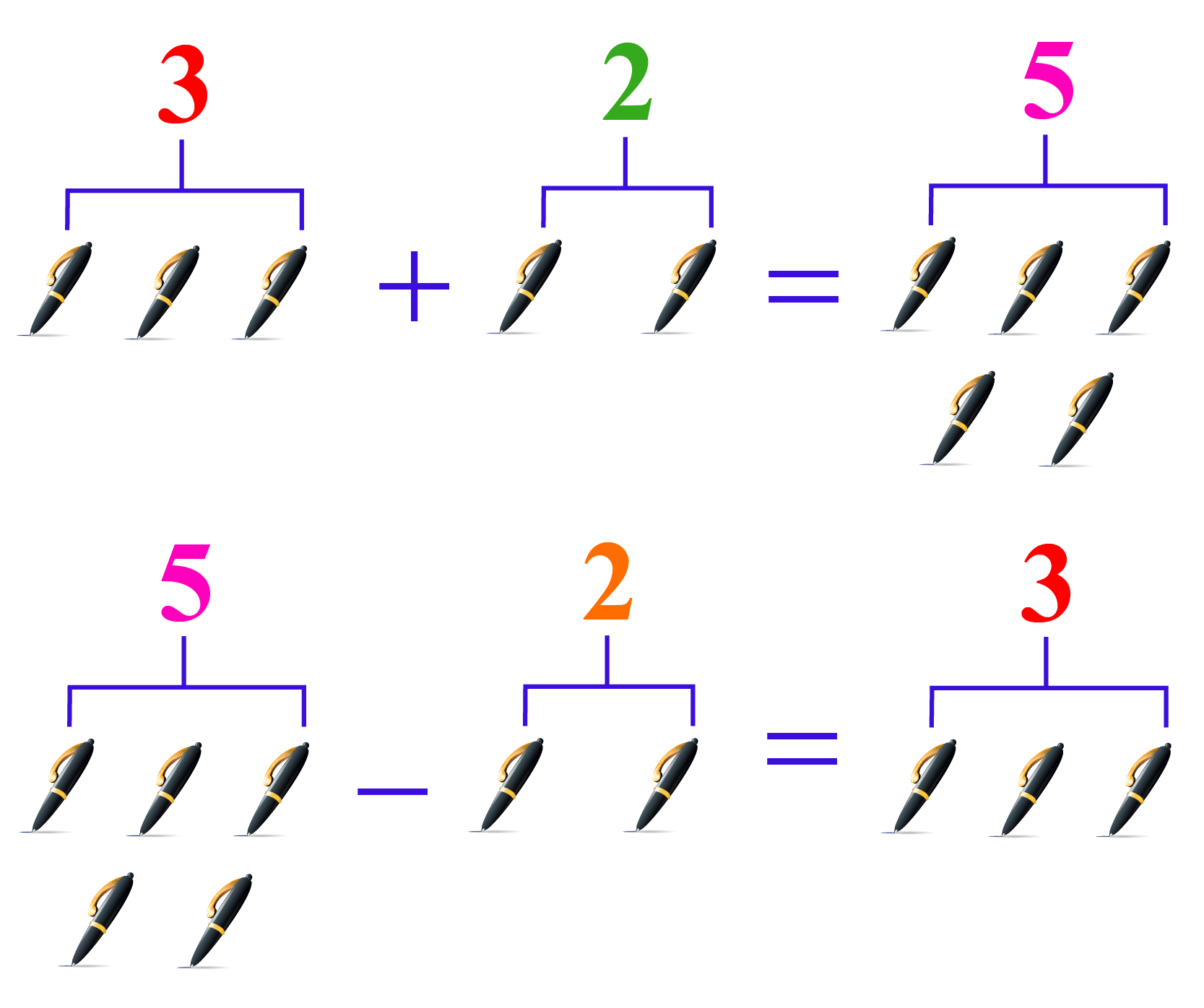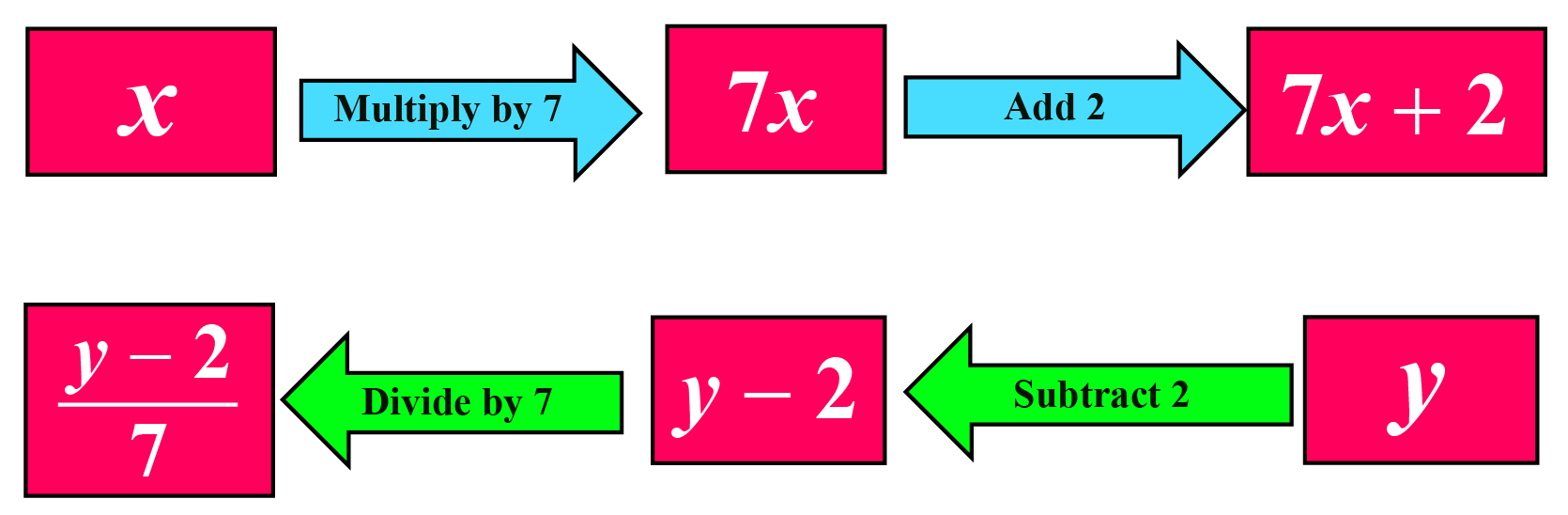# Inverse Operations

Inverse Operations

Have you ever wonder what would have happened if you were born before your elder sibling or vice-versa. Interesting, isn't it? Everything would have been inverted. Not in real life but it is possible in the world of math.

Let us consider here butterfly as an inverse of a honeybee.Would you expect this to happen in the real world?

In reality, it is not possible but mathematically we can prove so. Do you want to know how?

In this mini-lesson, we will explore the world of inverse operations by finding answers to questions like what are inverse operations, what are properties of inverses, and how to use inverse operations with help of interactive questions on inverse operations.

## Lesson Plan

 1 What Are Inverse Operations? 2 Important Notes on Inverse Operations 3 Solved Examples on Inverse Operations 4 Interactive Questions on Inverse Operations 5 Challenging Question on Inverse Operations

## What Are Inverse Operations?

Inverse means the opposite effect of an action or a step. In mathematics, we have operations such as addition$$(+)$$, subtraction$$(-)$$, multiplication$$(\times)$$, division$$(÷)$$, squaring, square root, and logarithms.

When we use two operations together, it is possible to have an inverse impact on the result due to the operations used.

The process in which the effect of one operation is inversed by another operation is termed as inverse operations.

For example,

If we add $$3$$ and $$2$$ pens we get $$5$$ pens, now subtract $$5$$ pens and $$2$$ pens and we get $$3$$ back. Here, addition and subtraction are inverse operations.## What Are the Properties of Inverses?

In the above section, we discussed that addition and subtraction are inverse operations. Now let us learn the properties of inverse operations in detail with few inverse operations examples.

### Property 1

The inverse of addition is subtraction. Addition always totals$$(+)$$ values and subtraction always takes away$$(-)$$ values.

For example, adding $$8$$ add $$2$$, we get $$10$$. Now, subtracting $$10$$ and $$2$$ and we get $$8$$ back.

There is also a property called additive inverse. In additive inverse, we add a certain value to a number (integer) to get zero as the final result.

For example,

$$-8 + 8 =0$$

$$-8$$ is an additive inverse of $$8$$

### Property 2

In inverse operations multiplication is the inverse of division. Multiplication can be undone with the help of division.

For example, multiplying $$8$$ $$\times$$ $$2$$ we get $$16$$, now divide $$16$$ $$\div$$ $$2$$ and we get $$8$$ back.

There is also a property called multiplicative inverse. In multiplicative inverse, we multiply a number by a certain fraction value $$(\dfrac{p}{q})$$ to get 1 as a final result.

For example,

$$8\times\dfrac{1}{8} = 1$$

$$\dfrac{1}{8}$$ is a multiplicative inverse of $$8$$

Note: Multiplicative inverse is not valid for zero.

For example, $$8\times0=0$$. It cannot be inversed by $$\dfrac{0}{0}$$.

### Property 3

In mathematics, the inverse of a function will always give us the original value.

For example, let's say hypothetically if the function f changes the butterfly into a honeybee then the inverse function $$f^{-1}$$ changes the honeybee back to the butterfly.Let's see a math example depicting the inverse of a function.

$$f(x)=7X + 2$$Here,

$$\dfrac{Y-2}{7}$$ is an inverse function of $$7X + 2$$.

### Property 4

The inverse of a trigonometry function.

Suppose that we apply the sine function to a certain angle $$\theta$$, and obtain the output as $$y$$. We can write

$y = \sin \theta$

This equation expresses $$y$$ in terms of $$\theta$$. Can we invert this relation to writing $$\theta$$ in terms of $$y$$?

Yes, we can invert the relation by writing

$\theta = {\sin ^{ - 1}}y$

Note: Do not confuse $${\sin ^{ - 1}}y$$ with $$\frac{1}{{\sin y}}$$. The latter is the reciprocal of $$\sin y$$. The former is completely different: the term  $${\sin ^{ - 1}}y$$  is to be interpreted as an angle value whose sine will be $$y$$.

The following table lists some examples of the $${\sin ^{ - 1}}$$ operation:

 $$\sin 0 = 0$$ $${\sin ^{ - 1}}0 = 0$$ $$\sin \frac{\pi }{6} = \frac{1}{2}$$ $${\sin ^{ - 1}}\left( {\frac{1}{2}} \right) = \frac{\pi }{6}$$ $$\sin \frac{\pi }{4} = \frac{1}{{\sqrt 2 }}$$ $${\sin ^{ - 1}}\left( {\frac{1}{{\sqrt 2 }}} \right) = \frac{\pi }{4}$$ $$\sin \frac{\pi }{3} = \frac{{\sqrt 3 }}{2}$$ $${\sin ^{ - 1}}\left( {\frac{{\sqrt 3 }}{2}} \right) = \frac{\pi }{3}$$ $$\sin \frac{\pi }{2} = 1$$ $${\sin ^{ - 1}}1 = \frac{\pi }{2}$$

Here are some examples of the $${\cos ^{ - 1}}$$ operation:

 $$\cos 0 = 1$$ $${\cos ^{ - 1}}1 = 0$$ $$cos\frac{\pi }{6} = \frac{{\sqrt 3 }}{2}$$ $$co{s^{ - 1}}\left( {\frac{{\sqrt 3 }}{2}} \right) = \frac{\pi }{6}$$ $$\cos \frac{\pi }{4} = \frac{1}{{\sqrt 2 }}$$ $$co{s^{ - 1}}\left( {\frac{1}{{\sqrt 2 }}} \right) = \frac{\pi }{4}$$ $$\cos \frac{\pi }{3} = \frac{1}{2}$$ $${\cos ^{ - 1}}\left( {\frac{1}{2}} \right) = \frac{\pi }{3}$$ $$\cos \frac{\pi }{2} = 0$$ $${\cos ^{ - 1}}0 = \frac{\pi }{2}$$

And a few examples of the $${\tan ^{ - 1}}$$ operation

 $$\tan 0 = 0$$ $${\tan ^{ - 1}}0 = 0$$ $$\tan \frac{\pi }{6} = \frac{1}{{\sqrt 3 }}$$ $${\tan ^{ - 1}}\left( {\frac{1}{{\sqrt 3 }}} \right) = \frac{\pi }{6}$$ $$\tan \frac{\pi }{4} = 1$$ $${\tan ^{ - 1}}\left( 1 \right) = \frac{\pi }{4}$$ $$\tan \frac{\pi }{3} = \sqrt 3$$ $${\tan ^{ - 1}}\left( {\sqrt 3 } \right) = \frac{\pi }{3}$$

### Property 5

Logarithm is an inverse of an exponent.

We express exponents in the form of power or degree $$3^2$$ here, $$2$$ is the exponent.

We can express the exponents in terms of the logarithm as:

$$3^2=9$$

$$\log_3(9)=2$$

Do you want to learn how to use inverse operations without using an inverse operations calculator? In our next section, we will learn the same.

## How to Use Inverse Operations?

Let us look at inverse operations examples to understand how to use inverse operations.

Example

We know that the inverse of f is $$f^{-1}$$ here we are going to use this relation in our inverse operations examples.

If $$x=5$$ solve $$f(x)=3x+3=18$$

$$f(5)=3\times5+3=18$$

$$f^{-1}(18)=\dfrac{18-3}{3}=5$$

Therefore,

$$f^{-1}(f(5))=5$$

Hence by applying function (f) and doing the inverese of the same function we are getting the original value back.

 $$f^{-1}(f(x)) = x$$ or $$f(f^{-1}(x)) = x$$

At Cuemath, our team of math experts is dedicated to making learning fun for our favorite readers, the students!

Through an interactive and engaging learning-teaching-learning approach, the teachers explore all angles of a topic.

Be it worksheets, online classes, doubt sessions, or any other form of relation, it’s the logical thinking and smart learning approach that we, at Cuemath, believe in.

## 1. What is the purpose of inverse operations?

Mathematically, inverse operations reverse the effect of one operation on the other. The main purpose is to understand the relation between the basic math operators $$+, -, \times, \div$$ so that solving an equation becomes easier and time saving.

## 2. What is the inverse operation of squaring a number?

The inverse of squaring a number is square root. For example, $$x^{2}=y$$ <=> $$\sqrt{y}=x$$ ($$x$$ $$≥$$ $$0$$).

## 3. What is the inverse operation of division?

In the inverse operations multiplication is the inverse operation of division.

For example, $$8$$ $$\div$$ $$2$$ we get $$4$$. Now multiply $$4$$ $$\times$$ $$2$$ and we get $$8$$ back.

More Important Topics
More Important Topics